# Disclosing hidden messages in a dim Star Light

Galileo by his telescope transformed Astrology into Astronomy in the modern sense and started the physical understanding of heavenly bodies. However, telescopes based on Geometrical Optics do not allow to see much at stellar distances. Further progress in the theory of light and its properties was needed for the study of stellar sizes, shapes and details such as star spots. This story is an elementary account of the physical principles that made such measurements possible and of some of the main results. I do include a few simple mathematical formulas, but people who prefer to skip them should be able to understand most of the story.

# Geometrical Optics and Physical Optics

Lenses were used in Roman times and Seneca described the magnifying power of a ball of crystal, but spectacles were invented much later, in 1290, by friar Alessandro Della Spina in Pisa. Moreover, only in 1608 the German spectacle maker Hans Lippershey in Holland tried to patent a telescope, possibly invented by someone else. Paolo Sarpi received a report on it; then his friend Galileo converted the news of a Dutch toy into a new scientific instrument capable of 30 magnifications. He made a series of amazing discoveries that he reported in Sidereus Nuncius.

This book started modern Astronomy and had a strong impact on our civilization even beyond Science. It also started the physical understanding of heavenly bodies, that according to Aristoteles did not consist of normal matter but of some perfect heavenly substance. It is surprising that inventing the telescope took so long, because it is based on simple properties of light rays and thin lenses. Since in the web there are several wrong descriptions in which light rays appear to change direction without reason, I wish to briefly explain the working principle of the astronomical telescope, to start with. The two properties of thin lenses that we need to understand the principle of an astronomical telescope are very simple. a) Light rays through the center of the lens go undeflected b) parallel light rays hitting the lens converge to a point called the focus of the lens. In Figure 1 two examples are shown. The left lens in Figure 1 is receiving an horizontal ray through the center, that is, along the axis; this continues without being deflected. It also receives two parallel rays from the left; they converge in the focus F of the lens. If the parallel rays are tilted the focus goes off-axis. The right lens in Figure 1 also receives an horizontal ray through the centre and a couple of parallel rays coming from the right that converge to the focus F.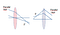Lenses receiving light from left and right.

These ideas are quite enough to understand the telescope. In order to get the principle of the astronomical telescope, we must simply flip over the right lens (with its rays), which then becomes the eyepiece.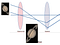Principle of the astronomical telescope

Now a light source in the far left in front of the objective lens would send a horizontal ray through the instrument, while a light source off the axis of the telescope would send the parallel rays coming from the left , which would converge to the focus of the objective, The instrument is built such that the focus of the eyepiece coincides with the focus of the objective. Consequently a couple of parallel rays emerge from the eyepiece and are ready to be observed since for the human eye the vision is optimal for parallel light rays; the image seen past the eyepiece is upside down, but one can set it upright by inserting a third lens, if necessary. The magnification of the image is obtained because the rays emerge from the eyepiece with a larger angle than the incident rays; to calculate the angular magnification, we must do a little elementary geometry as follows (Figure 3):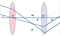Calculating the angular magnification of the telescope

Here, F is the common focus of both lenses, C and C’ are their centers; both angles α and β have been greatly exaggerated for graphical convenience, but must be considered as small, so CH is practically equal to the focal distance of the objective lens and HC’ is practically equal to the focal distance of the eyepiece; moreover to a good approximation and Therefore the image is amplified by a factor CH/HC’, that is the ratio of the focal lengths of the objective to the eyepiece. Since the focal length of the eyepiece cannot be less than a few centimeters, one must make long telescopes with long focal lengths of the objective. Accordingly, in the XVII long telescopes were built, but it was realized that at high magnifications one needed to collect much more light, otherwise the image was too dim and dark. Therefore the objective lens of the telescope must be big, too. And big lenses pose big practical problems. The sharpness of images is limited by chromatic aberration since the refraction index of the lens depends on the color of light, bur this trouble may be reduced by using achromatic lenses.

There are several variants of this instrument. The Galileo telescope had a diverging eyepiece and the Newton instrument collects light by a parabolic mirror which replaces the objective lens, but the basic principle of operation remains the same, namely, Geometrical Optics, which is the theory of light rays. Geometrical Optics also prompted the invention of microscopes and started new sciences, like microbiology. Thus, it was at the origin of a giant leap of Science.

Since the time of Padre Angelo Secchi, who introduced astronomical spectroscopy in the mid nineteenth century, star light brings information about the chemical composition of remote objects, showing that the same laws of Physics and Chemistry hold everywhere. Helium, unknown on Earth, was discovered on the Sun by spectroscopy.

Using the telescope James Bradley also discovered stellar aberration, that is fully explained by Relativity; later astronomers measured the immense distance of several nearby stars by a geometric method called parallax; this techniques consists of observing the tiny shift of the apparent position of the star in the course of the year. The star appears to move because of the Earth’s orbit, and using trigonometry its distance can be evaluated. The expansion of the Universe was discovered by Vesto Slipher and Edwin Hubble using spectroscopy. The large telescopes that allowed the discovery of galaxies and of the expanding Universe work basically on variations of the same principle as the Galileo telescope , which was developed in parallel with geometrical optics.

However, observing the stars by a telescope is disappointing: they appear as simple dots and the measurement of stellar shapes and diameters is not possible. For example, it is now known that Sirius (α Canis Majoris) has a diameter of 2.6 million Km and is one of the nearby stars, since its distance obtained by parallax is 8.6 light years. A light year is about 9.46.10 ¹⁵ m, a distance that we can hardly imagine. Thus the angular diameter is about 5 millionths of a radiant, and is below the detection limit of every telescope, including the Very Large Telescope. A related problem is that many stars have been found (mainly by spectroscopic methods) to be actually multiple systems that cannot be resolved by the present telescopes. According to the above presentation, the problem of improving the performance of the telescope appears to be geometrical. One could hope to reach any magnification by making the focal length of the objective very large, although this also required large diameters.

Nevertheless, it turns out that a fundamental problem arises from the wave nature of light. It was discovered by Young in 1801 that light does not really consist of rays but of waves. Indeed, the theory of Geometrical Optics is just an approximation, that can be justified in the limit when the wave lengths are negligibly short compared to the size of objects, slits and so on. In a telescopic image, one would like to appreciate sharp tiny features, but Fraunhofer diffraction, which arises from the wave length of light, sets a fundamental limit of sharpness. In the case of a telescope, the so-called Fraunhofer diffraction limits the angular resolution. If the objective lens has diameter D, the smallest angle that can be appreciated is

In order to see features on the surface of a star or to see how close binary stars orbit around their center of mass one should have an adequate angular resolving power but angles smaller than the above cannot be seen, and again one wants large D. Alas, the idea of building telescopes with larger and larger diameter D runs into technical and practical difficulties. Problems of weight, maneuverability and costs eventually become prohibitive. The Extremely Large Telescope with a compound mirror having D= 39 meters is being built and should start operating in 2024. However we are close to the limit of what can be achieved by Geometrical Optics. The project of the Overwhelmingly Large Telescope with a diameter of 100 meters aborted.

• At this point the only way to solve the problem is by following the famous statement by Roger Bacon: Natura non nisi parendo vincitur (The only way we can win over Nature is to obey its laws). Since light waves disobey Geometrical Optics, we must attain higher resolution by using the properties of light waves, that is, Physical Optics..

# The main idea of astronomy using waves , in short

Physicists and astronomers have found a different road to progress, which exploits in various nontrivial ways the knowledge of Physical Optics, that is, the science of light waves. A plane wave having wave length is characterized by a wave vector directed along the direction of propagation and having modulus

Thus, k summarizes the information about wave length and direction of propagation. Now let

denote the angle between the vectors of two waves emitted from different parts of the huge stellar surface and arriving to the pupil of an astronomer . For Sirius it turns out to be of the order of

and for most stars it will be even less. There is a different way to read equation (1-a), namely

In other terms, since the light waves coming from the star are not quite coming from the very same direction, there is a characteristic length D here on the surface of the Earth associated somehow with the size of a star. Smaller

implies larger D. But D is generally too large to be the diameter of a feasible telescope, and we must invent something else. This means that we must look for some other measurable characteristic magnitude f(x), where x is a distance on the Earth’s surface, related to starlight, with such a characteristic length D. We shall find that f(x) is simply a periodic function,

# The Young experiment and light waves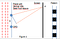Young experiment for normal incidence

More generally, if the monochromatic wave with wave length arrives at some angle (Figure 5) the OPD is modified and the pattern is simply shifted on the screen.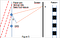Young experiment for off-normal incidence

In Figure 6, both plane waves of figures 4 and 5 with the same wave length impinge at different angles; but they are assumed to be incoherent, as they are if they arrive from two stars of a binary system or from different areas of the same star. In this case, each plane wave produces its own pattern and the two patterns simply sum. White and black areas superimpose in a random way. In Fig.6 the two patterns do not match. In this way, white becomes less white and black less black.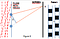Diffraction with two incoherent plane waves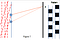Minimum V is attained for a proper spacing of the slits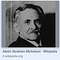Figure 8: Albert Michelson (Wikipedia)

One can realize this situation experimentally by closing the objective of a telescope by a diaphragm with two opposite holes; however, in this way S cannot be larger than the diameter of the objective. Fizeau  suggested to collect the light by separate mirrors, taking the light it to the telescope by means of other mirrors and letting it interfere there. The American physicist Albert Michelson developed the idea. Later Michelson became famous for the interferometer and the experiment that paved the way to the development of the Relativity Theory by Einstein. However for this subject a different Michelson interferometer is relevant.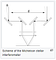Figure 9 The Michelson interferometer (from Wikipedia)

# Hanbury Brown and Twiss Effect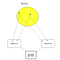Figure 10 Scheme of the Hanbury Brown and Twiss effect

Please note that in Fig.10 the distance between a and b may easily be of order one million kilometers, the dashed lines are several light years long while the D1-D2 distance D is of order one meter. So the Figure is completely out of scale. Both waves from a and b illuminate the two detectors D1 and D2; since the light waves fluctuate, the detectors produce fluctuating photocurrents . Actually, the points a and b are moving rapidly and randomly on the surface of the star. The waves are obviously incoherent, since the phases are random. Actually, even the number of points fluctuates randomly over time. Therefore, the detectors D1 and D2 generate time-dependent. fluctuating currents of intensities and . Both currents are sent to an electronic device, called correlator. The correlator produces the following output, which depends on the distance D between the two detectors:

Quantum mechanical aspects of the Hanbury Brown and Twiss effect

Although the effect is well described by the classical Maxwell equations, its quantum theory in terms of photons is also interesting. Different photons are emitted independently from different electrons which can be in distant parts of a large star. If one (correctly) conceives photons as independent quanta of light, but thinks of them (incorrectly) like of random bullets in a far west movie, no effect can be expected. The shower of bullets should be position independent, since nobody aims at one of the detectors; therefore, both detectors should receive a random shower of photons and no distance-dependent correlation between them would exist. Moreover, when both detectors receive a bullet, classically (at least in principle ) one can tell if it comes from a or from b.

But the quanta of light ( photons ) are indistinguishable Bosons and one cannot tell the origin of each of them. As a consequence of the Pauli spin-statistics theorem, the wave function of a pair of photons must be symmetric in their exchange, that is, nothing in the Universe can change when two identical photons are exchanged.

As Ugo Fano pointed out, one can understand the correlation by reasoning in terms of photon pairs. The photospheric electrons a and b emit the pair and the detectors 1 and 2 collect them. Then let A(a →1,b →2) denote the probability amplitude that the photon emitted by atom a goes to detector 1 and the photon emitted by atom b goes to detector 2, and A(a →2,b →1) the amplitude that the photons get exchanged; then the above mentioned rules of Quantum Mechanics prescribe that since the photons are Bosons, the probability amplitude of detecting a photon in 1 and a photon in 2 is

and the probability is the square modulus of the amplitude. For this reason, there is an enhanced probability of observing photons simultaneously at both detectors, a well known phenomenon known as photon bunching, which is familiar in Quantum Optics. Even if the Bosons do not interact, they tend to gather. By the same rules of Quantum Mechanics, when the particles are identical Fermions, the probability of detecting one in 1 and the other in 2 is the square modulus of

For this — sign, there is an reduced probability of observing Fermions simultaneously at both detectors, a quantum phenomenon known as antibunching; which has also been observed by Kimble et al. Using Na atoms in 1977. But this would take us too far. See Ref. 

## References

 M.Born and E. Wolf, Principles of Optics, Pergamon Press

 H. Fizeau, C.R. Acad. Sci. Paris, 66 (1868) 934

 Florentin Millour, New Astronomy Reviews 52 (2008)

Tabetha S. Boyajian et al., STELLAR DIAMETERS AND TEMPERATURES. I. MAIN-SEQUENCE A, F, AND G STARS

https://iopscience.iop.org/article/10.1088/0004-637X/746/1/101/pdf

M. Scholz, Stellar radii, SAO/NASA Astrophysics Data System 1997, http://articles.adsabs.harvard.edu/cgi-bin/nph-iarticle_query?bibcode=1997IAUS..189...51S&db_key=AST&page_ind=0&data_type=GIF&type=SCREEN_VIEW&classic=YES

 R.Hanbury Brown and R. Twiss, Nature 4497, 27 (1956);R.Hanbury Brown, ‘Measurements of stellar diameters’, Annual Reviews Inc. (1968) ans R. Hanbury Brown et al., Monthly Notices Roy. Astron. Soc. 137, 375 (1967)

 Gordon Baym,Lectures in Quantum Mechanics, Advanced book program

 T. Jeltes et al., ‘Comparison of the Hanbury Brown–Twiss effect for bosons and fermions’, Nature Letters 445 (2007)

Retired Full Professor, Tor Vergata University. Quantum Theory of Transport, Pumping, Magnetism, Superconductivity, Non linear Optics, Electron Spectroscopy,..

## More from Michele Cini

Retired Full Professor, Tor Vergata University. Quantum Theory of Transport, Pumping, Magnetism, Superconductivity, Non linear Optics, Electron Spectroscopy,..